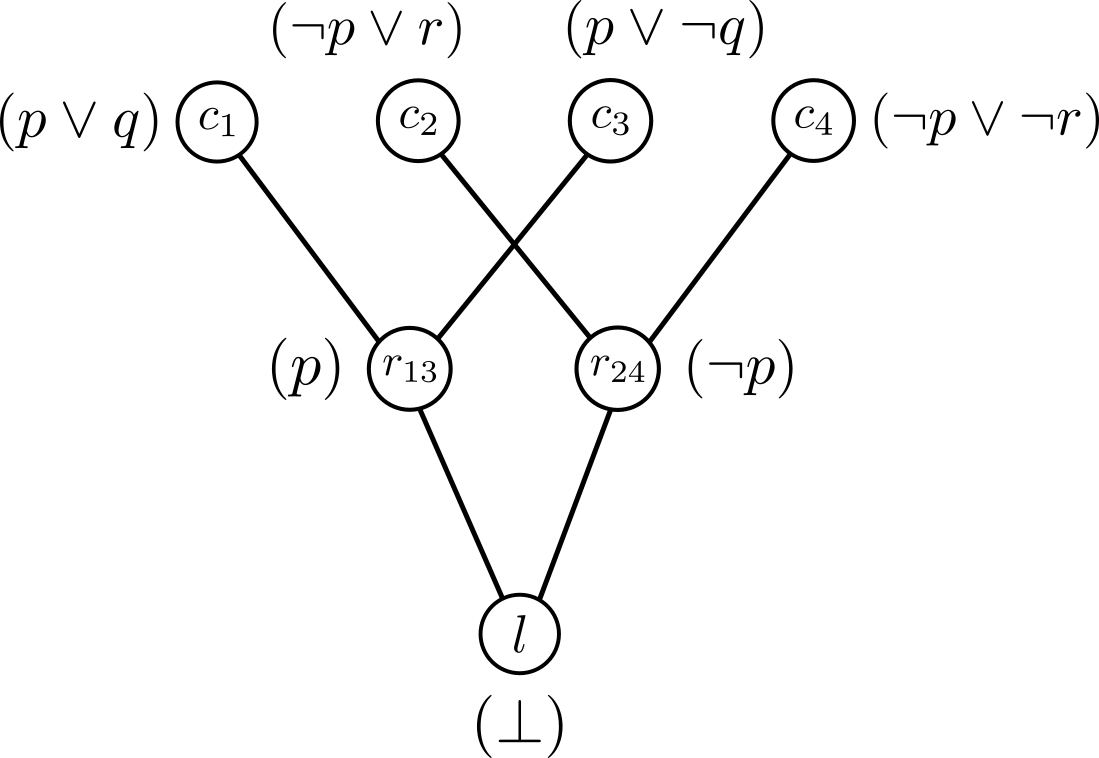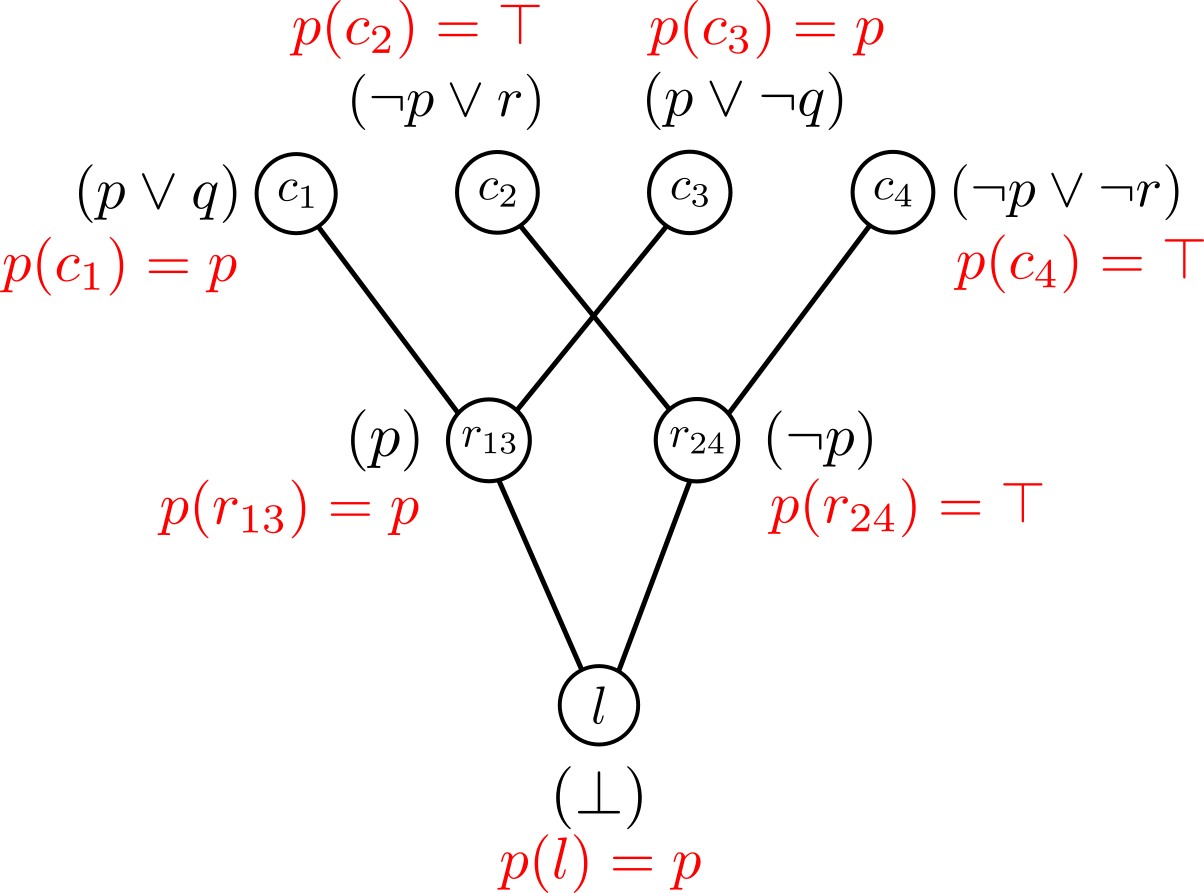# Interpolants and Relevance

• logic
• interpolants

» This post is a part of a series on ideas from symbolic logic lifted to verification «

For a pair of inconsistent formulas $(A, B)$, i.e., $A \wedge B \equiv \bot$ or unsatisfiable, the Craig Interpolant  for $(A, B)$ is a formula $\mathcal{I}$ such that

• $A \to \mathcal{I}$,
• $\mathcal{I} \wedge B \equiv \bot$, and
• $\mathcal{I}$ contains variables common to $A$ and $B$.

The interpolant can be viewed as the part of $A$ that is sufficient, or relevant, to contradict $B$. Modern SAT solvers produce a proof of unsatisfiability, or resolution proof, if the checked formula is unsatisfiable. An interpolant can be extracted in linear time  from a proof of unsatisfiability. The chief advantage of deriving interpolants from proofs is that they capture facts which the SAT solver derived about $A$ in showing that $A$ is inconsistent with $B$. Therefore, assuming that the prover ignores irrelevant facts and focuses on real ones, interpolation can be thought of as a way of filtering out irrelevant information from $A$ .

## Interpolants from Proofs

Preliminaries. A literal is a Boolean variable or its negation. A clause is a disjunction of zero or more literals. A clause set is satisfiable if there exists a truth assignment to the Boolean variables that makes all clauses in the set true. Given two clauses $c_1 = a \vee b$ and $c_2 = \neg a \vee c$, the resolvent of $c_1$ and $c_2$ is the clause $b \vee c$, assuming $b \vee c$ is non-tautological, and $a$ is the pivot variable. Similarly, $a \vee b$ and $\neg a \vee \neg c$ have resolvent $b \vee \neg c$, while $a \vee b$ and $\neg a \vee \neg b$ have no resolvent, since $b \vee \neg b$ is tautological. Therefore, the resolvent of clauses $c_1$ and $c_2$ is a clause that is implied by $c_1 \wedge c_2$, and $\exists v (c_1 \wedge c_2)$, where $v$ is the pivot variable.

### Proof of Unsatisfiability

A proof of unsatisfiability $\Pi$ for a set of clauses $C$ is a directed acyclic graph $(V, E)$, where $V$ is a set of clauses, and

• for every vertex $c \in V$, either
• $c \in C$, and $c$ is a root, or
• $c$ has exactly two predecessors, $c_1$ and $c_2$, such that $c$ is their resolvent, and
• the empty clause is an unique leaf.

If a proof on unsatisfiability exists for a set of clauses, then the clause set is unsatisfiable. In the satisfiable case, a SAT solver produces a satisfying assignment, whereas in the unsatisfiable case, it can produce a proof on unsatisfiability. The Wikipedia article on Resolution, along with Propositional Resolution, is a good starting point on the use of resolution to check satisfiability of a set of clauses.

Example 1: Consider clause set $C = \{c_1, c_2, c_3, c_4\}$, such that $c_1 = (p \vee q)$, $c_2 = (\neg p \vee r)$, $c_3 = (p \vee \neg q)$, and $c_4 = (\neg p \vee \neg r)$. The proof of unsatisfiability graph for $C$ is shown in Figure 1. $r_{13}$ is resolvent of clauses $c_1$ and $c_3$, and $r_{24}$ is resolvent of $c_2$ and $c_4$. Resolvent clauses $r_{13}$ and $r_{24}$ are resolve to the empty leaf clause. Therefore, $C$ is unsatisfiable.Figure 1: A proof of unsatisfiability graph for clauses (p \vee q), (\neg p \vee r), (p \vee \neg q), and (\neg p \vee \neg r).

### Algorithm to find Interpolants

Suppose we are given a pair of clause set $(A, B)$ and a proof of unsatisfiability $\Pi$ of $A \wedge B$. Some terminology:

• Global variable: a variable appearing in both $A$ and $B$.
• Local variable: a variable appearing only in $A$.

Similarly, a literal is global or local to $A$ depending on the variable it contains. Given a clause $c$, $g(c)$ denotes the disjunction of the global literals in $c$, and $l(c)$ denotes the disjunction of local literals in $c$ with respect to $A$.

Example 2: We have two clauses, $c_1 = (a \vee \neg b \vee c)$ and $c_2 = (\neg a \vee b \vee \neg d)$, and suppose $A = \{c_1\}$ and $B = \{c_2\}$. Then

• $g(c_1) = (a \vee \neg b)$,
• $l(c_1) = (c)$,
• $g(c_2) = (\neg a \vee b)$, and
• $l(c_2) = (\bot)$.

Algorithm: The description of the algorithm to find interpolant from proofs is based on . Let $(A, B)$ be a pair of set clauses and $\Pi = (V, E)$ be the proof of unsatisfiability graph for inconsistency of $A \wedge B$. For every vertex $c \in V$, we compute a boolean formula $p(c)$ as follows.

if c is root, i.e. c ∈ V then
if c ∈ A then
p(c) = g(c)
else
p(c) = True
else
a, b = predecessors of c
v = pivot variable of a, b
if v is local to A then
p(c) = p(a) ∨ p(b)
else
p(c) = p(a) ∧ p(b)


The interpolant of $(A, B)$ is $p(c = \bot)$, where $c$ is leaf vertex in $\Pi$, or $p(\bot)$. The interpolant for $(A, B)$ is not unique and depends on the proof of unsatisfiability $\Pi$. Therefore, we often denote interpolant for $(A, B)$ with respect to $\Pi$ as $ITP(\Pi, A, B)$.

Complexity: Let $N$ be the number of vertices in $\Pi$, i.e., $N = |V|$, and $L$ be the number of literals appearing in $\Pi$, i.e., $L = \Sigma_{c \in V} |c|$. It the worst case, lines 2–5 repeat $N$ times, and lines 7–8 repeat $L$ since every literal in $\Pi$ can be a pivot variable. Therefore, the formula $ITP(\Pi, A, B)$ can be computed in $O(N + L)$ time, i.e., linear in the size of $\Pi$.

Example 4: Consider the clause set $C = \{c_1, c_2, c_3, c_4\}$, such that $c_1 = (p \vee q)$, $c_2 = (\neg p \vee r)$, $c_3 = (p \vee \neg q)$, and $c_4 = (\neg p \vee \neg r)$, from Example 1. The proof of unsatisfiability for $C$ is shown in Figure 1. Let $A = \{c_1, c_3\}$ and $B = \{c_2, c_4\}$. We know that $A \wedge B$ is unsatisfiable. we first find the global and local literals for each clause in $C$: $g(c_1) = (p)$, $l(c_1) = q$, $g(c_2) = \neg p$, $l(c_2) = \neg q$, $g(c_3) = p$, $l(c_3) = \bot$, $g(c_4) = \neg p$, $l(c_4) = \bot$. Figure 2 shows the $p(c)$ formulas computed using the above algorithm for every $c$ vertex of Figure 1.Figure 2: Intermediate p(c) formulas for every vertex c in the proof of unsatisfiability graph of Figure 1.

For the leaf vertex $l$, $p(l) = p$. Therefore, $ITP(\Pi, A, B) = p$. It can be verified that $\mathcal{I} = p$ is the interpolant of clause sets $(A, B)$ since $A \to \mathcal{I}$, $\mathcal{I} \wedge B \equiv \bot$, and $\mathcal{I}$ contains variables common to both $A$ and $B$.

1. K L McMillan. 2005. Applications of Craig Interpolants in Model Checking. In 11th International Conference on Tools and Algorithms for the Construction and Analysis of Systems (TACAS), 1–12.
2. K L McMillan. 2003. Interpolation and SAT-Based Model Checking. In 15th International Conference on Computer Aided Verification (CAV), 1–13.
3. Pavel Pudlák. 1997. Lower bounds for resolution and cutting plane proofs and monotone computations. The Journal of Symbolic Logic 62, 3 (1997), 981–998.
4. William Craig. 1957. Linear reasoning. A new form of the Herbrand-Gentzen theorem. The Journal of Symbolic Logic 22, 3 (1957), 250–268.